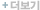### 문헌홈 > 저널 > 상세

#### 한국생활환경학회|한국생활환경학회지 2002년 12월

기사명 생체전기임피던스법에 의한 한국성인여성의 제지방량 추정공식 / Prediction Equation for Estimating Body Composition from Bioelectrical Impedance Method for Korean Women 김현수 한국생활환경학회 한국생활환경학회지 , v.9 n.4(2002-12) 시작페이지(290) 총페이지(6) 1226-1289 기타 ; BIA ; FFM ; Underwater-weighing ; Validity ; Prediction Equation The bioelectrical impedance analysis (BIA) has been proposed as a convenient, valid approach to estimate body composition in normal, healthy people. BIA prediction equations are based on either population-specific or generalized models. The aims of this study were to develop a convenient, useful equation that would sufficiently predict fat free mass (FFM) and to determine the validity of predict equation in Korean women. The participants were 220 Korean women aged 18~59 years (height 159.2±5.2 cm, body weight 58.5±7.3 kg; means±sd). FFM based on underwater weighing (UW) was used as the criterion for validity. To construct prediction equations for FFM, we used multiple regression analysis, whereby all possible combinations were examined. The linear multiple regression equation constructed for predicting the FFM was, for instance, FFM = 22.444 + 0.00444*(BW H2/R) - 0.0944*age - 0.257*T, where, BW = body weight (kg), H: body height (cm), R = impedance (ohms), T = triceps skinfold thickness (mm). Four equations developed in the present study were then cross-validated in different sample (n = 27) of women. The results indicate that the above equation had the lowest SEE (2.38 kg). The FFM calculated using the new equations did not differ from that based on the UW method. In conclusion, the prediction equations in this study afford accurate and inexpensive means of estimating FFM in Korean adult women without having them remove their clothing. 한국생활환경학회 한국어

• 375건 다운
• 260건 다운
• 226건 다운
• 200건 다운
• 143건 다운
• 123건 다운

## 최근 등록 논문## 최근 등록 저널